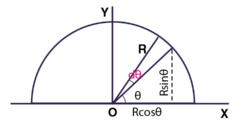# Find the center of mass of uniform semicircular ring of radius R and mass MThe wire is uniform, the mass per unit length of the wire is

$\frac{M}{\pi R^{2}}$

The mass of the element is

$dm = \left ( \frac{M}{\pi R} \right )\left ( R d\theta \right )$

$dm = \left ( \frac{M}{\pi } \right )d\theta$

The coordinates of the center of mass are

$X = \frac{1}{M}\int xdm = \frac{1}{M}\int_{0}^{\pi }\left ( R cos\theta \right )\left ( \frac{M}{\pi } \right )d\theta = 0$

$X = \frac{1}{M}\int ydm = \frac{1}{M}\int_{0}^{\pi }\left ( R sin\theta \right )\left ( \frac{M}{\pi } \right )d\theta = \frac{2R}{\pi }$

Therefore, position of centre of mass i

$\left ( 0, \frac{2R}{\pi } \right )$